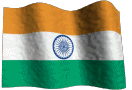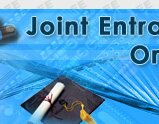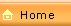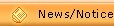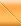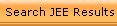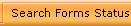Introduction Availability of Courses Eligibility Criteria Syllabi (with sample ques) Application Procedure Inst for filling Form Admit Card Rules for JEE-06 Examination Centres Intake Capacity Reservation of Seats Admission Rules Fees Structure Contact Us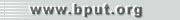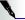MCA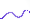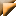MATHEMATICSLogicStatement, Negation, Implication, Converse, Contra positives, Conjuction, Disjunction, Truth Table.Algebra of SetsSet operations, Union, Intersection, Difference, Symmetric Difference, Complement, Venn Diagram, Cartesian products of sets, Relation and Function, Composite Function, Inverse of a Function , Equivalence Relation, Kinds of Function.Number SystemsReal numbers (algebraic and other properties, rational and irrational numbers), Complex numbers, Algebra of complex numbers, Conjugate and square root of a complex number, cube roots of unity, De-Moivre’s Theorem with simple application. Permutation and combinations and their simple applications, Mathematical induction, Binomial Theorem. Determinants upto third order, Minors and Cofactors, Properties of determinants. Matrices upto third order, Types of Matrices. Algebra of matrices, Adjoint and Inverse of a matrix. Application of determinants and matrices to the solution of linear equations (in three unknowns).TrigonometryCompound angles, multiple and Sub-multiple angles, solution of trigonometric equations, Properties of triangles, Inverse circular function.Co-ordinate Geometry of Two DimensionsStraight lines, Pairs of straight lines, Circles, Equations of tangents and normals to a circle. Equations of Parabola, Ellipse and Hyperbola, Ellipse and hyperbola in simple forms and their tangents (Focus, directix, eccentricity and latus rectum in all cases).Co-ordinate Geometry of Three DimensionsDistance and division formulae, Direction cosines and direction ratios. Projections, Angles between two planes, Angle between a line and a plane, Distance of a point from a line and plane. Equations of a sphere-general equation.VectorsFundamentals, Dot and Cross product of two vectors, Scalar triple product, Simple Applications (to geometry, work and moment).Differential CalculusConcept of limit, Continuity, Derivation of standard functions, successive differentiation simple cases, Leibnitz Theorem, Partial differentiation Simple cases, derivatives as rate measure, Maxima and minima indeterminate forms, Geometrical applications such as tangents and normals to plane curves.Intergal CalculusStandard methods of integration (substitution, by parts, by partial fractions etc.). Definite integrals and properties of Definite Integrals, Areas under plane curves, Differential Equations ( only simple cases.).1 dy/dx = f (x) 3. d2y/dx2= f (x) and applications to motions in a straight line with constant acceleration. 2 dy/dx = f (x) .g(y)Probability and StatisticsAverages (Mean, Median and Mode), Dispersion (standard deviation and variance). Definition of probability, Mutually exclusive events. Independent events, Addition theorem.COMPUTER AWARENESSIntroduction to ComputerBrief history of Computers, Components of a Computer, Computer related general knowledge, Application of Computers, Classification of Computers, Simple DOS Commands. Computer Arithmetic: Number System with general base, Number base conversion, Elementary arithmetic operation.BASIC Language ProgrammingFlow Charts, Algorithms, Constants, Variables, Arithmetic and logical expression, Elementary BASIC statements, Writing simple programs (using sequence, repetition and control structures), subscripted Variables, Matrix operations Function and Subroutines, Concept of Files.NOTE: The Question will cover the entire course and will be multiple choice type similar to the ones given in Section 7.5.